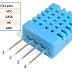DHT11 digital temperature and humidity sensor is a composite Sensor contains a calibrated digital signal output of the temperature and humidity. Today in this tutorial we will be using DHT11 sensor with STM32.

## INITIALIZATION

As you can see above, in order to initialize the sensor, we have to pull the data line LOW for around 18 ms. After this DHT11 will pull the line low for 80us and than high for 80us. Once this is done, the sensor will be initialized and start transmitting
NOTE:- You need to connect pull-up resistance to the data line or else DHT11 will not be able to pull it HIGH.
##### So to initialize the sensor, steps are as follows:-
1.)  Set the pin (data) as output.
2.) Pull the pin low and wait for 18ms.
3.) set the pin as input for receiving the data.
DHT11 will now send the response as you can see in the figure above.
##### To check the response, steps are as follows:-
1.) wait for 40us
2.) check if the pin is low, than wait for 80us. This will totally be a delay of 120us and the pin should be high now.
3.) Check if the pin is high. If it is, than the response is ok.
4.) Now wait for the pin to go low as you can see below that the pin is low for 50us before transmitting any bit.

Now DHT11 will send 40 bits of data.  Each bit’s transmission begins with low-voltage-level that last 50us, the following high-voltage-level signal’s length decides whether the bit is “1” or “0”.
So if the length of high-voltage-level is around 26-28us, the bit is “0”.
And if the length is around 70us, than the bit is “1”.
The 40 bits sent by DHT11 are as follows DATA = 8 bit integral RH data + 8 bit decimal RH data + 8 bit integral T data+8 bit decimal T data + 8 bit checksum
If the data transmission is right, check-sum should be the last 8 bit of “8 bit integral RH data+8 bit decimal RH data+8 bit integral T data+8 bit decimal T data”
##### Reading data is also very simple. Steps are as follows:-
1.) Wait for the pin to go high.
2.) Wait for 40us. This is because the length of “0” bit is 26-28us  and if the pin is high after 40us, it indicates that the bit is “1”.
3.) write the respective values to the variable.

## Some Insight into the CODE

##### INITIALIZATION
1.)  Set the pin (data) as output.
2.) Pull the pin low and wait for 18ms.
3.) set the pin as input for receiving the data.
``````void DHT11_start (void)
{
set_gpio_output ();  // set the pin as output
HAL_GPIO_WritePin (GPIOA, GPIO_PIN_1, 0);   // pull the pin low
DWT_Delay_us (18000);   // wait for 18ms
set_gpio_input ();   // set as input
}
``````
##### RESPONSE
1.) wait for 40us
2.) check if the pin is low, than wait for 80us. This will totally be a delay of 120us and the pin should be high now.
3.) Check if the pin is high. If it is, than the response is ok.
``````void check_response (void)
{
DWT_Delay_us (40);
if (!(HAL_GPIO_ReadPin (GPIOA, GPIO_PIN_1)))  // if the pin is low
{
DWT_Delay_us (80);  // wait for 80us
if ((HAL_GPIO_ReadPin (GPIOA, GPIO_PIN_1))) check = 1;  // now if the pin is high response = ok i.e. check =1
}
while ((HAL_GPIO_ReadPin (GPIOA, GPIO_PIN_1)));   // wait for the pin to go low
}
``````
1.) Wait for the pin to go high.
2.) Wait for 40us. This is because the length of “0” bit is 26-28us  and if the pin is high after 40us, it indicates that the bit is “1”.
3.) write the respective values to the variable.
``````
{
uint8_t i,j;
for (j=0;j<8;j++)
{
while (!(HAL_GPIO_ReadPin (GPIOA, GPIO_PIN_1)));   // wait for the pin to go high
DWT_Delay_us (40);   // wait for 40 us
if ((HAL_GPIO_ReadPin (GPIOA, GPIO_PIN_1)) == 0)   // if the pin is low
{
i&= ~(1<<(7-j));   // write 0
}
else i|= (1<<(7-j));  // if the pin is high, write 1
while ((HAL_GPIO_ReadPin (GPIOA, GPIO_PIN_1)));  // wait for the pin to go low
}
return i;
}``````

How to use DHT11 Temperature sensorReviewed by arun singh on July 06, 2018 Rating: 5